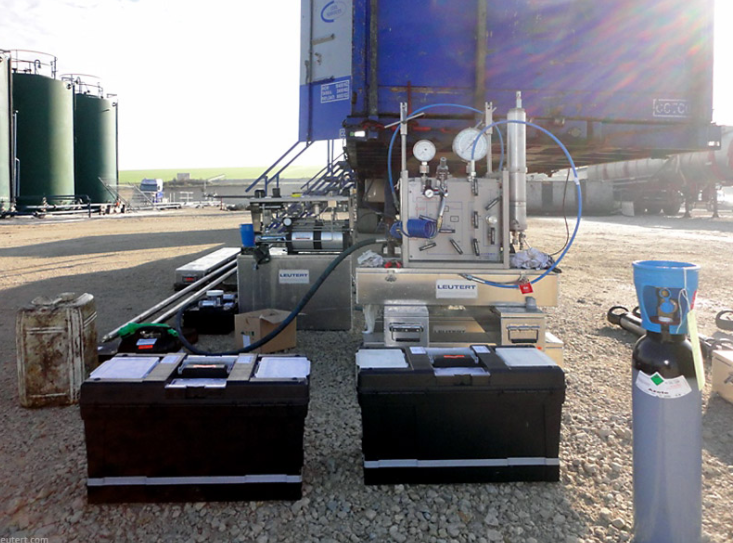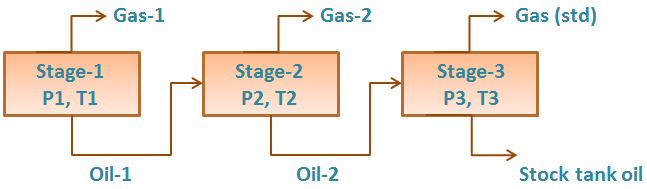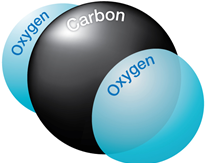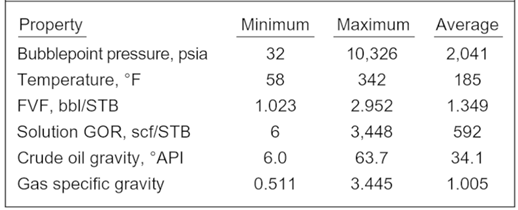PVT Sampling Methods: Downhole, Wellhead & Surface Recombination

Samples of the reservoir fluid are usually collected at an early stage in the well’s producing life and dispatched to a laboratory for the f PVT analysis. The objectives of sampling are to receive samples from a suitable place in the production wells or surface facilities. The samples should represent the system in the reservoir under its initial conditions in order to determine its type, volumetric and phase behavior, and its composition. PVT analysis results are needed for geological and reservoir engineering evaluation and forecasting, as well as for laboratory studies concerning enhanced oil recovery (EOR).There are basically three ways of collecting fluids samples from a well, either by Downhole SamplingWellhead Sampling or by  Surface Recombination Sampling.

PVT Experiments: Separator Test

Separator Test experiments are carried out for both oil and gas condensate mixtures. A sample of reservoir liquid is placed in the laboratory cell and brought to reservoir temperature and bubble-point pressure. Then the liquid is expelled from the cell through a number of stages of separation.  Usually, two or three stages of separation are used, with the last stage at atmospheric pressure and near-ambient temperature (60 to 80°F).

The gas is let out of the separator through the top and is transferred to standard conditions, where its volume is measured. As for the differential liberation experiment, liquid dropping out from the gas is converted to an equivalent gas volume at standard conditions.

The liquid from the ﬁrst separator is let into a second separator at a lower pressure and temperature than the ﬁrst one. At which conditions, more gas will be liberated as sketched in the figure below. As with the gas from the ﬁrst separator, this gas is transferred to standard conditions.The oil remaining after gas removal is brought to the conditions of the next separator stage. The gas is removed again and quantified by moles and specific gravity. Oil volume is noted, and the process is repeated until stock-tank conditions are reached. Final oil volume, Vo, and specific gravity, SGo, are measured at 60°F.

Effect of CO2 on downhole flowrate calculation

Downhole flow rate can be calculated from surface flow rate (stock tank barrels) using the following equation. It is assumed that no gas is dissolved in the water phase and the water formation volume factor is equal to one.

Downhole flow rate = [(Oil rate)sc × Bo] + [(Free GOR) × (Oil rate)sc × Gas FVF] + (Water rate)sc

Free GOR = Producing GOR – Solution GOR, therefore:

q = ( Qo × Bo ) + [ ( R – Rs) × Qo × Bg × 1000] + Qw

Where:

• q = downhole flow rate (bbl/d or m3/d)
• Qo = Oil flow rate at standard conditions (stb/d or m3sc/d)
• Bo = Oil formation volume factor (bbl/stb or m3sc/m3sc)
• R = Producing gas-oil ratio (scf/stb or m3sc/m3sc)
• Rs = Solution gas-oil ration (scf/stb or m3sc/m3sc)
• Bg = Gas formation vol. factor (bbl/mscf or m3sc/m3sc)
• Qw = Water flow rate at standard conditions (stb/d or m3sc/d)

Effect of CO2 on downhole flowrate calculation:If CO2 is present, the calculation of downhole flow rate becomes more complex for many reasons:

PVT Properties and Correlations

Ideally, laboratory measured PVT data should be utilized. Many times, laboratory data is not available and correlations must be used instead. This post will discuss PVT properties and correlations that can be used to estimate them. It is difficult to say which correlation should be used when. This is because most of the correlations were developed with regional crude samples. The best correlation is the one that matches your data.Many investigators have used PVT laboratory test results, and field data, to develop generalized correlations for estimating properties of reservoir fluids. The main properties which are determined from empirical correlations are the bubble point, gas solubility, volume, density, compressibility, and viscosity. The correlations typically match the employed experimental data with an average deviation of less than a few percent. It is not unusual, however, to observe deviations with an order of magnitude higher when applied to other fluids.

1. Bubble Point Pressure (Pb):

The bubble point pressure, also known as the saturation pressure, is the pressure, at some reference temperature, that the first bubble of gas is liberated from the liquid phase. The reference temperature is usually the reservoir temperature, but any temperature can be used. Note that the bubble point pressure is a function of temperature and changing the reference temperature will change the bubble point pressure.

Statistical analysis of correlations:

Al-Shammasi, in his SPE paper “A Review of Bubble point Pressure and Oil Formation Volume Factor Correlations” (SPE-71302-PA, April 2001), compiled a databank of 1,243 data points from the literature. This was supplemented by 133 samples available from a GeoMark Research database (GeoMark Research. 2003. RFD base (Reservoir Fluid Database)), bringing the total number of data points to 1,376. These data were then used to rank the bubble point pressure correlations. The following Table summarizes the ranges of data used for bubble point pressure, temperature, oil FVF, Solution GOR, oil gravity, and gas specific gravity.